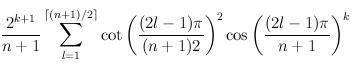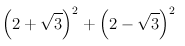## Friday, February 01, 2008

### Cool formula

Pi comes up in the most unexpected places. Here's an application to walk counting that involves it.

Suppose you have a chain of length n. How many walks of length k are there on the chain? For instance, for a chain of length 5, there are 5 paths of length 0 (start at each vertex and don't go anywhere), 8 of length 1 (traverse each edge either left-right or right-left), 14 of length 2 (8 walks that change direction once, 6 walks that don't change direction) etc.

Curiously enough, there's an explicit formula for thisFor example, to find the number of walks of length 2 in a chain of length 5, plug n=5, k=2 into formula above, and getWhich is 14.

Notebook, web version

Zeppe said...

:D
that formula is amazing! Could you put a reference to it, please? I'm really curious to see the demonstration :)Anonymous said...

this is a very interesting blog. I will surely go back again.

http://www.artificiallife.bitsystemsph.com

Yaroslav Bulatov said...

Zeppe, I got the formula from p.214 of Spectra of Graphs: Theory and Applications (scanned copy)

Number of walks of length n is the sum of entries of A^k where A is the adjacency matrix of the graph. From that you could guess that the formula would have something to do with eigenvalues of the adjacency matrix.

For instance, the number of self-returning walks of length k is the trace of A^k, which is e1^k+e2^k+.... where e's are eigenvalues of the adjacency matrix of the graph

I'm still working out the mechanics of finding eigenvalues for matrices like this, but they seem to come up pretty often in physics. For instance, see page 5 of http://arxiv.org/abs/cond-mat/0001408, it gives expression for eigenvalues of the adjacency matrix of a loop of length n. Using expression from eq.24 there you would get a simple formula for number of self-returning walks of length k in a loop

Yaroslav Bulatov said...

"Number of walks of length n is the sum of entries of A^k", should be "Number of walks of length k is the sum of entries of A^k"

draj said...

Excellent machine learning blog,thanks for sharing...
Seo Internship in Bangalore
Smo Internship in Bangalore
Digital Marketing Internship Program in Bangalore

john said...
john said...